4. Mineral Materials:  Investigation 4.2

# How can we measure the volumes of rocks?

## Plan Investigation 4.2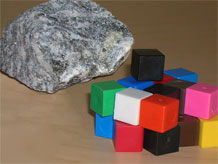The students are getting good at measuring different kinds of earth materials. They can weigh rocks and minerals and cubes with ease using the digital scale. They can measure the volume of liquid samples using their own "measuring cups." But how can they measure the volume of an irregularly shaped solid, like a key or a rock or a handful of sand?

### Formative Assessment

Do students understand that a collection of centimeter cubes equal to the volume of a rock will displace an equal amount of water?

Available online at inquiryproject.terc.edu

A clue can be found in the last investigation. Students discovered that when a rock is submerged in water, it displaces a "rock's worth" of volume. In this investigation, students find a way to measure that volume in cubic centimeters. By the end of the investigation students will understand that the volume of solid objects can be found by measuring the amount of water they displace.

### Learning Goals

• Recall that volume is measured in cubic centimeters
• Understand that the volume of a solid object can be found by water displacement
Sequence of experiences
1. Ask the question All Class 15 Mins
2. Estimate and measure the volume of rocks Small Groups 15 Mins
3. Share the volume data Small Groups 5 Mins
4. Discuss the results Discussion 10 Mins

### Materials and Preparation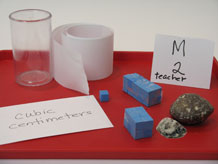For the class:

• Post the investigation question in a place where all students can see it.
• 1 classroom volume measure line (see kit for instructions)
• 1 12cc rectangular block made by joining 12 centimeter cubes in a 2x2x3 shape (use clear tape).
• 1 20cc rectangular block made by joining 20 centimeter cubes in a 2x2x5 shape (use clear tape).
• 1 centimeter cube
• 1 empty 150cc container
• 2 rocks or minerals of different sizes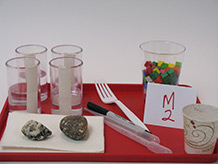For each tray:

• 2 rocks or minerals
• 1 cup holding approximately 75 centimeter cubes
• 4 150cc containers each with a vertical strip of masking tape applied to the outside, approximately half full of water
• 1 3oz cup of water
• 2 pipettes
• 1 fine tip permanent marker
• 2 paper towels
• 1 plastic fork

### Notebook Pages

Formative Assessment

## Do students understand that a collection of centimeter cubes equal to the volume of a rock will displace an equal amount of water?

For evidence, observe students as they work, listen to their conversation with peers, ask questions, e.g., “Would you tell me about what you are doing?”

To interpret the evidence, ask yourself; Does this student:

• Begin with equal volumes of water?
• Compare the water level of the rock and cubes and note that when the water levels are equal, the number of cubes are equal to the volume of the rock?
• Count the number of cubes and describe the volume of the rock in cubic centimeters?

A possible next step would be to ask students to use props or drawings to describe what they did, why they did it, and what they found out.

All Class 15 MinsMeet in a circle and show students the two rocks or minerals.

• Which rock has the greater volume? How much greater?

Listen to the students’ comparisons. Do they distinguish weight from volume? Can they express relative volume ("This one is twice as big"; "That one has less stuff in it"?) Do they remember the correct unit of measure — cubic centimeters? Do they suggest submerging the rocks in water to find out? (If yes, tell them to "Hold that thought!") As you field the comments, encourage students to think about a quantitative comparison:

Weight vs. volume:  Fourth graders sometimes have difficulty distinguishing weight and volume. If you hear this confusion entering the discussion, stop and ask, "Are you talking about weight or volume? If this rock were made of Styrofoam or feathers instead of minerals, would its volume be any different? No, volume is the amount of space the rock takes up, not how much it weighs."

• What if we want to know exactly how much greater, how could we figure that out?

Estimating volume:  Thinking about how much space objects take up can sometimes be easier when we see those objects enclosed in defined spaces — like a plastic container. If students are having difficulty imagining solid volumes, take a moment to practice estimation skills.

Place each of the three demonstration blocks inside an empty 150cc container, one at a time, starting with the largest. Ask, "How many more of these 20cc [12 cc, 1cc] blocks do you think can fit into this container?" Four to six 20cc blocks will fit comfortably in the container, and it will hold more than six 12cc blocks.

You might also measure the volumes of some small, common classroom objects before class, and make them available for reference during the demonstration.

Draw attention to the investigation question:

How can we measure the volumes of rocks?

Let students brainstorm some ideas. Entertain all ideas for now.

Bring out the three blocks — the 1cc cube, the 12cc block, and the 20cc block — and pass them around. Ask students to inspect each one carefully, looking from all sides and counting the number of centimeter cubes, then ask for the volume of each object (1cc, 12cc, 20cc).

Volume vs. surface area:  Fourth graders will sometimes confuse volume with surface area, counting the two-dimensional "faces" of the cubes on the outside of the block instead of the three-dimensional cubes that make up its volume. Model the three-dimensional space with your hands or take one of the blocks apart, count the individual cubes with the class, then tape the cubes back together again.

Bring out the volume line and ask a volunteer to place the three blocks on the line. Point out that the volume line is just like a weight line, e.g. it starts at zero and has even intervals of measure, but it is marked in cubic centimeters and is used to compare the volumes of objects. Ask volunteers to place the two rocks on the volume line where they estimate they should go.

Does the volume line help us to see any more information about how the volumes of the objects compare?

Sum up the work so far. Students have estimated the volume of the two rocks using their eyes and three reference blocks. But they still don't have a measured volume.

Can we get any closer to the actual volumes of the two rocks? Is there any way we can use our centimeter cubes to help us?

Demonstrate how to estimate the volume of a rock by replicating its size and shape with a pile of centimeter cubes. Then, place two 150cc containers, approximately half full of water, side by side with the cubes in front of one container and the rock in front of the other.

If we want to find out if this replica has the same volume as the rock, what could we do?

Listen for ideas about water displacement and settle on a procedure like this one:

1. Equalize the amount of water in the two containers, using the pipette
2. Place the rock in one container and add the cubes to the second container, counting as you go, until the water levels are the same.

## 2. Estimate and measure the volume of rocks

Small Groups 15 Mins Notebook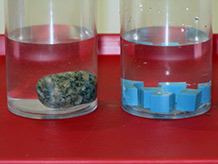Distribute a tray of materials to each group. Explain that each pair of students will estimate and then measure the volume of one of the rocks on the tray; they will then combine their data with the data for the second rock collected by the other pair.

Students estimate the volume of their rock. They first build a rock replica using centimeter cubes. Students record their estimates in their notebooks specifying the unit of measurement (cubic centimeters) each time [Which rock has more volume?].

Students then check their estimates using the water displacement procedure devised by the class, recording the procedure and results in their notebooks.

Steps for Measuring the Volumes of the Rocks:

1. Equalize the starting water levels and mark them on the containers.
2. Place the rock in one container and mark the new water level after the water has been displaced.
3. Add centimeter cubes to the second container, one at a time, counting as you go, until the water level matches the water level in the first container. The number of cubes is the measured volume.

## 3. Share the volume data

Small Groups 10 Mins Notebook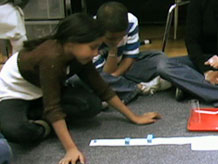After the pairs finish their measurements, the students fill out the group's data table in their notebooks. They then place their rocks on the class volume line, labeling each rock with the measured volume. Finally, they record the position of their rocks on the volume line in their notebooks [Measured volumes].

## 4. Discuss the results

Discussion 10 Mins Notebook

### Purpose of the discussion

The purpose of the discussion is for students to be able to explain how to measure the volume of rocks and other solid objects, and understand the advantages of using displacement to determine volume. Again, focus the discussion on the investigation question.

### Engage students in the focus question

To engage students in the discussion, first allow time for them to complete the Reflection Questions in their notebooks [Volume reflection], and then return to the investigation question:

How can we measure the volumes of rocks?

• If you wanted to find the volume of your hand, would you use cubic centimeters to build a replica or use displacement of water? Why?

If there is time, ask students to consider the following question:

Do you think the displacement method for measuring solid volumes would work if we used mineral oil instead of water? Why? (Yes, any liquid will work.)

Let students know that it is often difficult to estimate volume, just as it can be difficult to estimate weight. Practice helps, but measurement is better. Unfortunately, there is no "volume scale" like a "weight scale." For irregularly shaped objects, we can measure volume only indirectly, by measuring water displacement, as the students did today.

### Summarize the discussion and recap the investigation

Use students' own words to summarize how can we measure the volume of rocks and other objects using displacement.

As you review the investigation, make sure students understand the following main points:

• Volume is often measured in cubic centimeters.
• With practice, we can estimate volumes by comparing new objects with objects whose volumes are already known.
• The volume of any solid objects can be measured by the displacement of water.